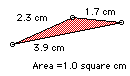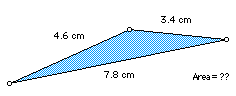# Biggie Size It (Triangle)If you double the lengths of each of the sides of a triangle, what happens to the perimeter and the area? Explain why.

How would the results change if you triple the lengths of each of the sides? What if you make the sides ten times their original size? Explain your reasoning.Extension:
If you double the area of a triangle, explain what happens to the side lengths and perimeter.

By how many times would you need to increase the area in order for the new side length and new perimeter to be 5 times the original measures?